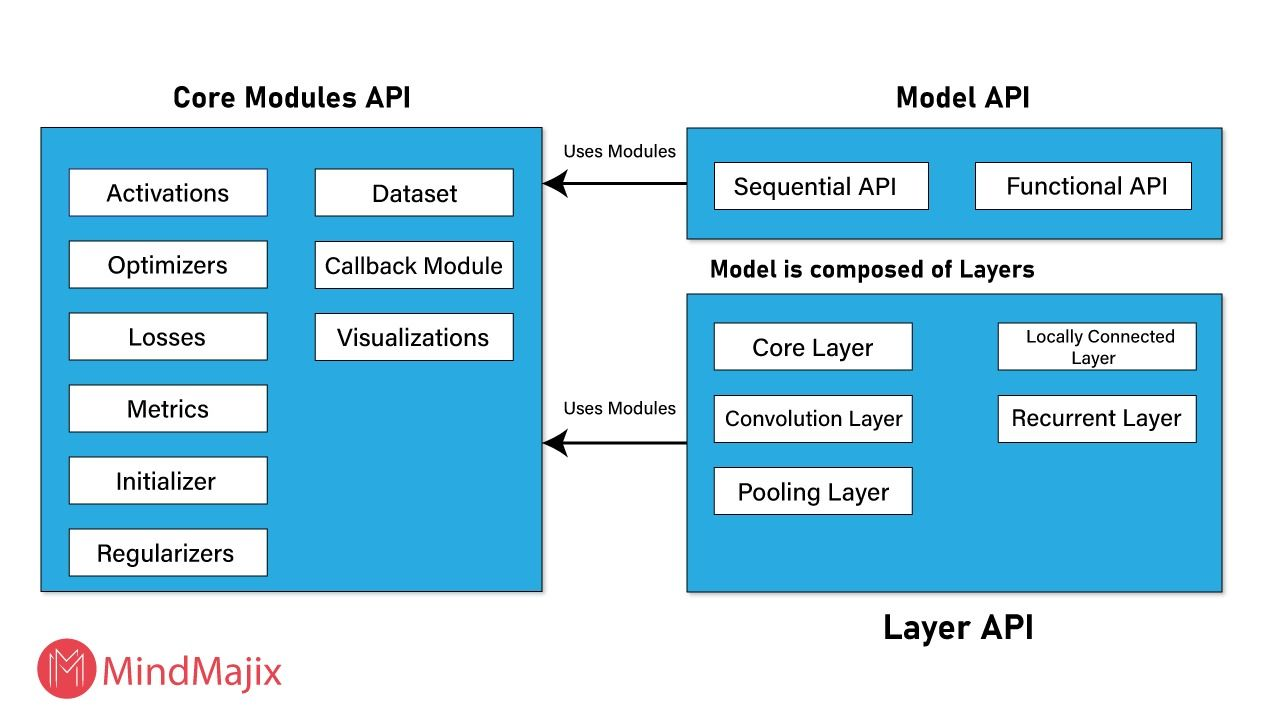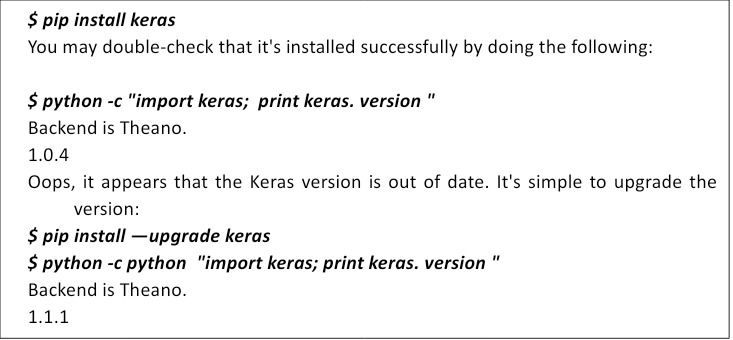# Complete Keras Tutorial: Deep Learning in PythonKeras is a Python-based, open-source deep learning framework. It runs on top of TensorFlow, a machine learning platform. The brainchild of Francois Chollet, a Google artificial intelligence researcher, Keras is presently used by big names like Google, Square, Netflix, Huawei, and Uber.

This article will cover the basics of deep learning, Keras installation, its models, layers, modules, and applications.

## What is Keras?

Keras is a human-centric API that was created to allow for quick experimentation. It adheres to best practices for lowering the cognitive load, such as providing uniform and simple APIs, limiting the number of user activities required for typical use cases, and providing clear and actionable error messages. It comes with a lot of documentation and developer instructions.

## Why is it called Keras?

Keras is a Greek word that means ‘horn’. It's a reference to a literary image from ancient Greek and Latin literature, first found in Homer's Odyssey. Here, dream spirits (Oneiroi, singular Oneiros) are divided into those who manipulate dreamers with false visions - arriving through an ivory gate - and those who reveal a future that will come to pass - arriving through a horn gate. Keras was created as part of the ONEIROS (Open-ended Neuro-Electronic Intelligent Robot Operating System) project's research effort.

## Features of Keras

Keras has several features that make it a leading deep learning API.

• It’s consistent, easy to use, and expandable.
• It can iterate as quickly as you can think.
• It enables machine learning on an exascale.
• It can be used anywhere.
• It has a massive ecosystem.
• It can enable cutting-edge research.
• It’s a framework that works on both the CPU and the GPU.

## Architecture of Keras

Keras has three main modules: model, layers, and core modules.

### 1. Models

Keras models are divided into two categories:

Sequential model

Keras layers are arranged in a certain order. All of the known neural networks may be represented using the sequential model.

Functional API

This aids in the creation of complex models.### 2. Layers

Each Keras layer in the Keras model corresponds to the corresponding layer in the actual neural network model. The following are the fundamental Keras layers:

• Stacking layers
• Layers of convolution
• Core layers
• Recurrent layers

### 3. Core modules

These are some of the neural network functionalities provided by Keras:

- Activations module: This module provides several activation functions such as relu, softmax, etc.
- Optimizer module: This contains optimizer functions such as sgd, adm, and others.
- Regularizers: They offer the L 1 Regularizer and L 2 Regularizer routines.
- Loss module: This contains loss functions such as Poisson, mean absolute error, etc.

## Keras for deep learning

Machine learning includes deep learning as a subdomain. Deep learning's fundamental task is to examine the input layer by layer. The following are some of the foundations of deep learning:

### 1. Artificial Neural Network (ANN)

Artificial neural networks are the most used deep learning approach. The human brain model is what drives them. Just as there are interconnected neurons in the human brain, there are interconnected nodes in constructing hidden layers in ANNs.

The nodes in the inner layers will analyze the supplied input, which will pass via further hidden layers before reaching the output layer and anticipating the result. For example, the output layer could provide the desired result.

### 2. Multilayer perceptron (MLP)

This is the simplest kind of ANN. It has one input layer, several hidden layers, and one output layer in the end. In a multilayer perceptron, one hidden layer processes a portion of the input and sends it to the other hidden layer. A single neuron or several neurons can be found in each buried layer. The data is then sent to the output layer by the last concealed layer. Finally, the output layer produces the desired result.

### 3. Convolutional neural network (CNN)

CNN is a well-known artificial neural network. It is widely utilized in video and image recognition applications, and is based on the notion of ‘convolution’ in mathematics. It is the same as a multilayer perceptron. Its major layers are as follows:

- Convolution layer: A fundamental element that performs arithmetic operations using the convolution function.
- Pooling layer: Located next to the convolution layer, its purpose is to lower the input size by removing redundant data.
- Fully connected layer: Organizes data into multiple categories and sits alongside the pooling and convolution layers.

### 4. Recurrent neural network (RNN)

RNNs are neural networks that are used to find other ANN models' flaws. The primary function is to save previous data and choices. This approach is mostly used to categorize images. In addition, the bidirectional RNN is beneficial for predicting the future based on the past.

## How to install Keras

If you have used Anaconda, you will already have pip installed, which is a package management system. You can check this by running \$ pip on the command prompt. It should return a selection of commands and alternatives to choose from.You can now use Keras.

## Keras for Naive users

1. Import libraries and modules

`import numpy as np`
`np.random.seed(123) # for reproducibility`

`from keras.models import Sequential`
`from keras.layers import Dense, Dropout, Activation, Flatten`
`from keras.layers import Convolution2D, MaxPooling2D`
`from keras.utils import np_utils`
`from keras.datasets import mnist`

2. Load pre-shuffled MNIST data into train and test sets

`(X_train, y_train), (X_test, y_test) = mnist.load_data()`

3. Preprocess input data

`X_train = X_train.reshape(X_train.shape, 1, 28, 28)`
`X_test = X_test.reshape(X_test.shape, 1, 28, 28)`
`X_train = X_train.astype('float32')`
`X_test = X_test.astype('float32')`
`X_train /= 255`
`X_test /= 255`

4. Preprocess class labels

`Y_train = np_utils.to_categorical(y_train, 10)`
`Y_test = np_utils.to_categorical(y_test, 10)`

5. Define model architecture

`model = Sequential()`

`model.add(Convolution2D(32,3,3,activation='relu', input_shape=(1,28,28)))`
`model.add(Convolution2D(32,3,3, activation='relu'))`
`model.add(MaxPooling2D(pool_size=(2,2)))`
`model.add(Dropout(0.25))`

`model.add(Flatten())`
`model.add(Dense(128, activation='relu'))`
`model.add(Dropout(0.5))`
`model.add(Dense(10, activation='softmax'))`

6. Compile model

`model.compile(loss='categorical_crossentropy',`
`optimizer='adam',`
`metrics=['accuracy'])`

7. Fit model on training data

`model.fit(X_train, Y_train,`
`batch_size=32, nb_epoch=10, verbose=1)`

8. Evaluate model on test data

`score = model.evaluate(X_test, Y_test, verbose=0)`

## Keras application

Keras applications are deep learning models that come with weights that have already been trained. Prediction, feature extraction, and fine-tuning are all possible with these models. When you create a model, the weights are downloaded automatically. Keras is a powerful platform with industry-leading performance and scalability. As it relieves developers’ cognitive load, it enables focus on the most important aspects of a problem. Simple workflows should be quick and easy, but arbitrarily advanced workflows should be achievable via a clear path that builds on what one has already learned. And this is where Keras helps.### Press

What's up with Turing? Get the latest news about us here.### Blog